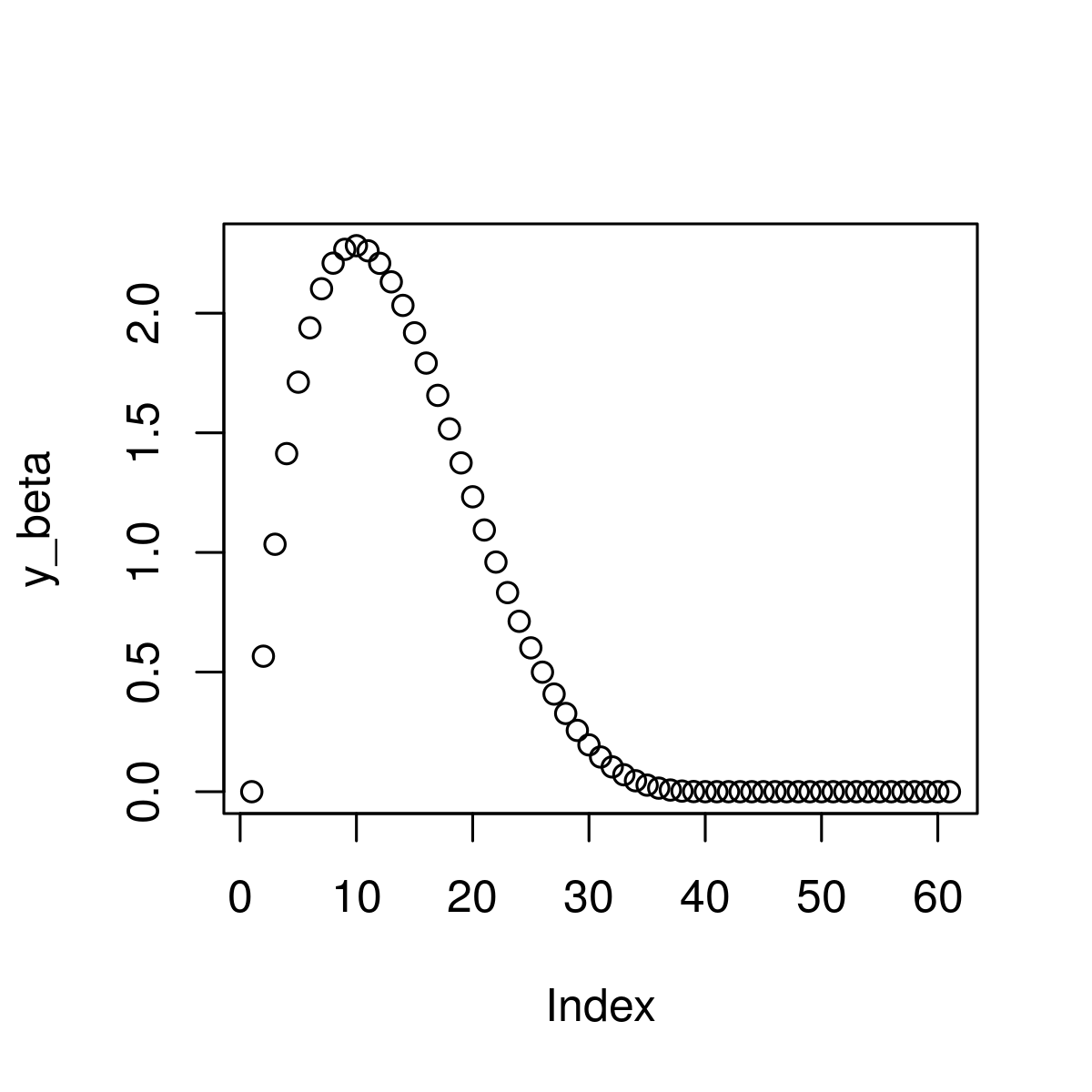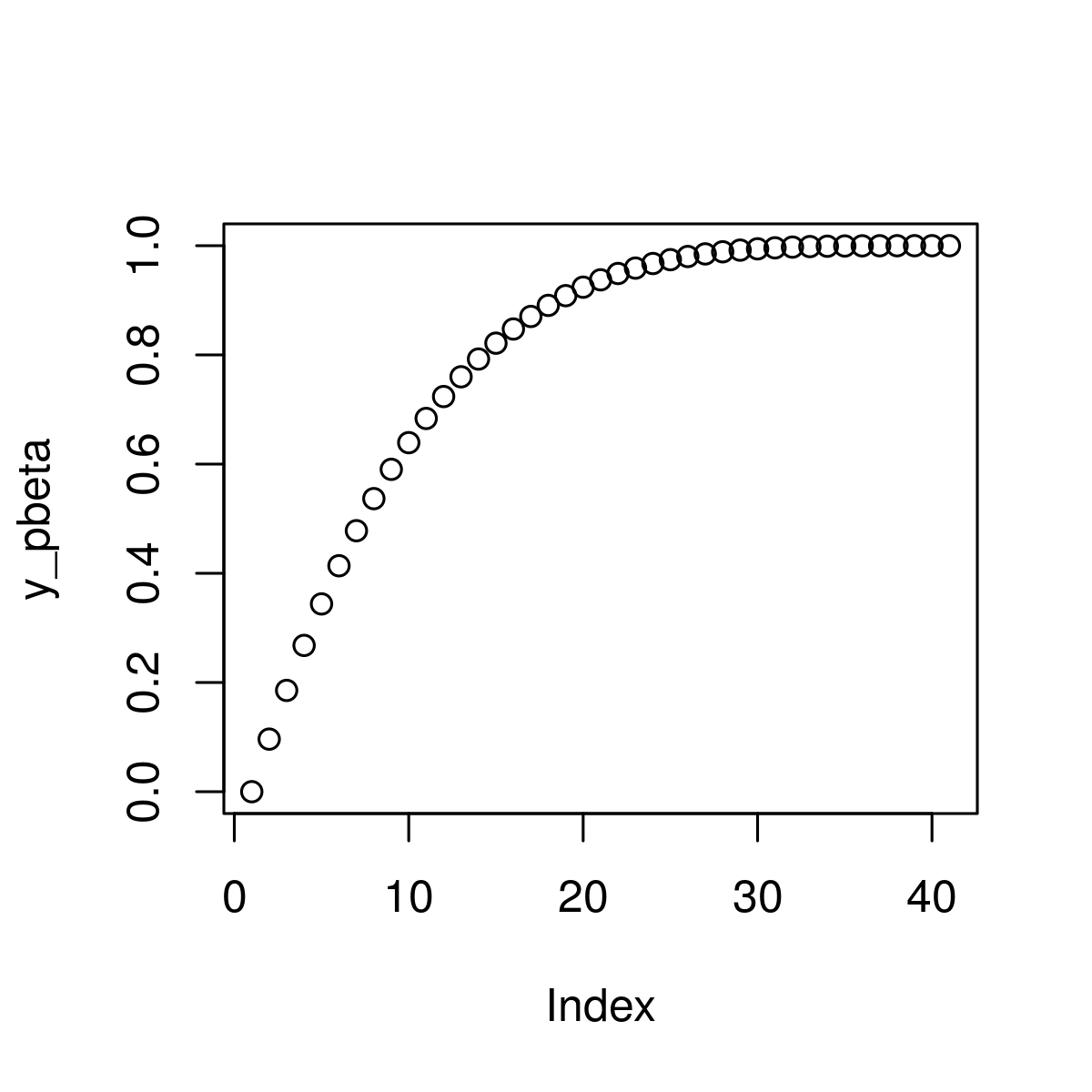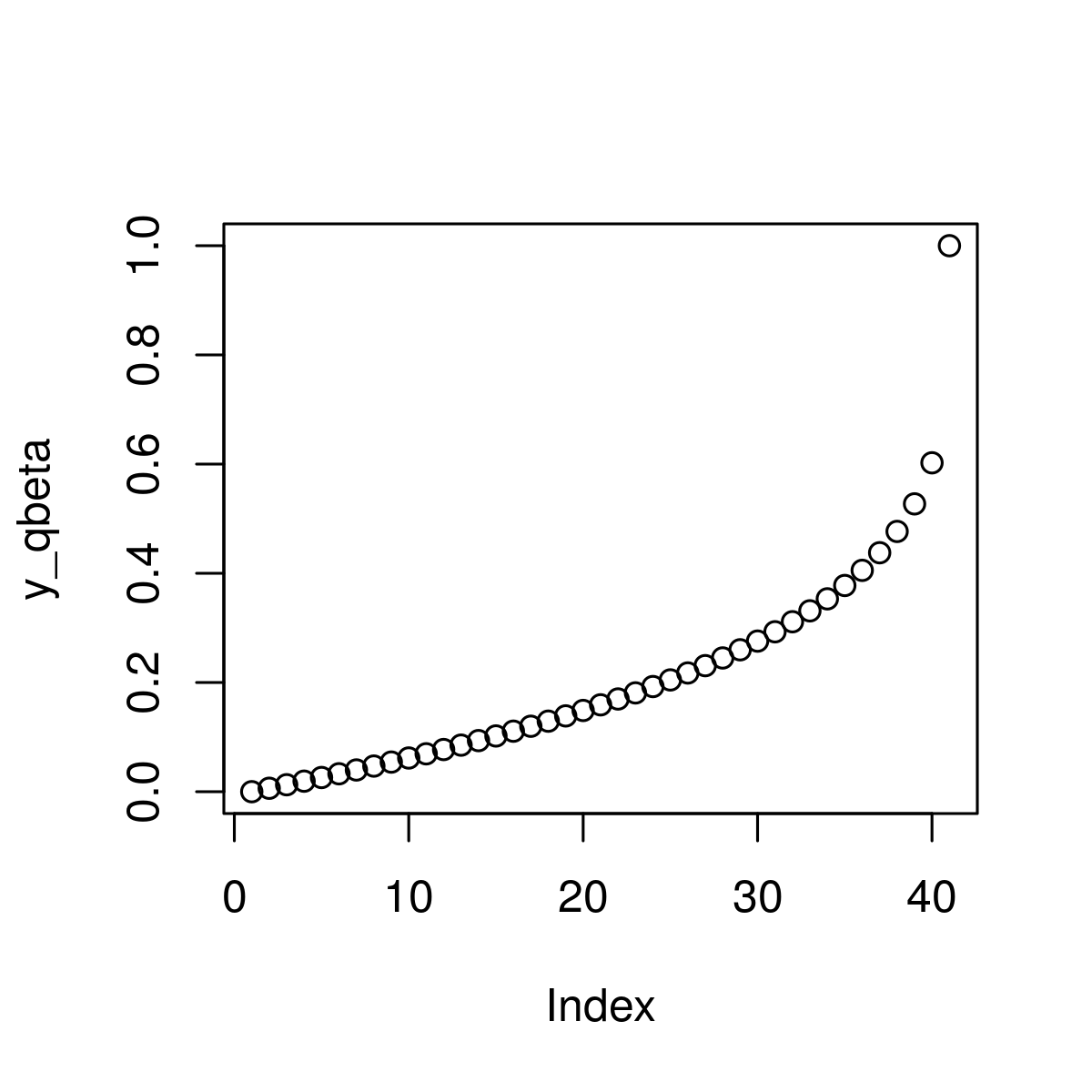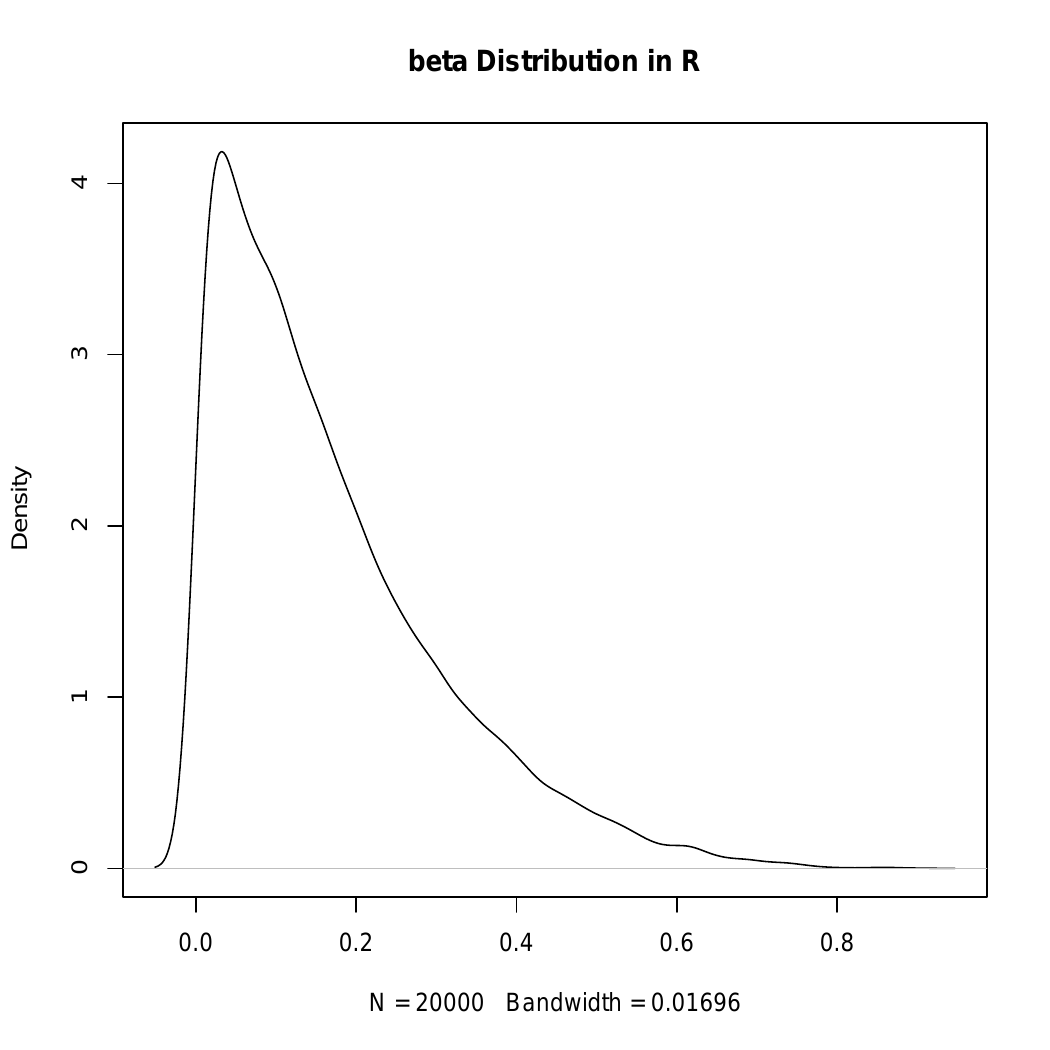Open in App
Not now

# Compute Beta Distribution in R Programming – dbeta(), pbeta(), qbeta(), and rbeta() Functions

• Last Updated : 19 Dec, 2022

Beta Distribution in R Language is defined as property which represents the possible values of probability. This article is an illustration of dbeta, pbeta, qbeta, and rbeta functions of Beta Distribution.

#### dbeta() Function

It is defined as Beta Density function and is used to create beta density value corresponding to the vector of quantiles.

Syntax: dbeta(vec, shape1, shape2) Parameter: vec: Vector to be used shape1, shape2: beta density of input values Returns: beta density values for a vector of quantiles

Example :

## r

 `# R program to illustrate the use of ` `# dbeta() function`   `# Creating a vector` `x_beta <- ``seq``(0, 1.5, by = 0.025 )  `   `# Apply beta function          ` `y_beta <- ``dbeta``(x_beta, shape1 = 2, shape2 = 4.5)  `   `# Plot beta values` `plot``(y_beta)                                       `

Output:#### pbeta() Function

It is used to create cumulative distribution function of the beta distribution.

Syntax: pbeta(vec, shape1, shape2) Parameter: vec: Vector to be used shape1, shape2: beta density of input values

Example:

## r

 `# Specify x-values for pbeta function` `x_pbeta <- ``seq``(0, 1, by = 0.025)      `   `# Apply pbeta() function` `y_pbeta <- ``pbeta``(x_pbeta, shape1 = 1, shape2 = 4)  `   `# Plot pbeta values` `plot``(y_pbeta)`

Output:#### qbeta() Function

It is known as beta quantile function and used to return quantile values of the function.

Syntax: qbeta(vec, shape1, shape2) Parameters: vec: Vector to be used shape1, shape2: beta density of input values

Example:

## r

 `# Specify x-values for qbeta() function` `x_qbeta <- ``seq``(0, 1, by = 0.025)`   `# Apply qbeta() function` `y_qbeta <- ``qbeta``(x_qbeta, shape1 = 1, shape2 = 4)  `   `# Plot qbeta() values` `plot``(y_qbeta) `

Output:#### rbeta() Function

It is defined as a random number generator that is used to set seed and specify sample size.

Syntax: rbeta(N, shape1, shape2 ) Parameters: vec: Vector to be used shape1, shape2: beta density of input values

Example:

## r

 `# Set seed for reproducibility` `set.seed``(13579)`   `# Specify sample size` `N <- 10000  `   `# Draw N beta distributed values` `y_rbeta <- ``rbeta``(N, shape1 = 1, shape2 = 5)   ` `y_rbeta`   `# Plot of randomly drawn beta density` `plot``(``density``(y_rbeta), ` `     ``main = "beta Distribution ``in` `R")`

Output:My Personal Notes arrow_drop_up
Related Articles